# EECS 545: Homework 3

## 1) Cross-validated (batch) ridge regression (20pts)

### a)

(5 pts) We will use the 1st variable (CMEDV) of the 14 variables as our response y and the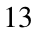remaining variables as the feature variables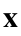. Sphere the train-validate feature variables, i.e., transform the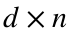feature matrix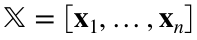so that its rows have zero sample mean and sample variance equal to one (see Slide 17 of Lec 3 ). Display the vector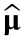of sample means and the vector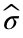of sample standard deviations. These vectors should be 13 dimensional and you will use them to likewise transform the test data.
clear
clc
y = boston.CMEDV;
X = transpose(table2array(boston(:,2:end)));
[d, n] = size(X);
subset = 460;
[X_sph, mu, sigma] = sphere_X(X(:, 1:subset));
disp(mu)
3.6731 10.9359 11.1597 0.0696 0.5547 6.2818 68.6800 3.7950 9.6674 409.4587 18.4759 357.2528 12.6795
disp(sigma)
8.7132 22.8004 6.8092 0.2547 0.1147 0.7053 27.8636 2.0960 8.7768 169.6645 2.1781 91.5471 7.1469

### b)

(5pts) Here you will be using the using the Sphered train-validate data produced in part (a). Using all the data except for the hold-out sample compute the effective degrees of freedom (Lec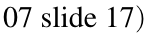over the range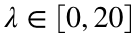with a step size 0.1 and plot the result. For this same range of λ find the ridge regression coefficients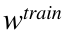that minimize the PRSS over the train-validate sample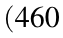instances) and plot its mean squared prediction errorsas a function of λ. Now apply your learned predictor without regularization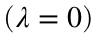to the test samples in the hold-out set and report its mean squared prediction error. Note: to be applied to a novel instance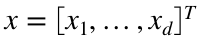in the test set, you need to transform the novel sample the same way you transformed the training sample when learning the predictor coefficients. In particular, with whain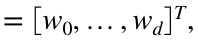the predictor on the novel sample will have form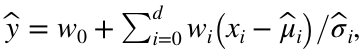where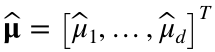and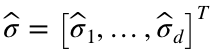were found in part a.
step = 0.1;
N = 20 / step;
lambdas = linspace(0, 20, N + 1);
MSE = zeros(N + 1, 1);
EDD = zeros(N + 1, 1);
w_train = zeros(d + 1, subset);
for i = 1:(N + 1)
% Parameters
lambda = lambdas(i);
X_til = X_sph(:,1:subset);
y_T = y(1:subset);
[d, ~] = size(X_til);
% Compute w_hat, y_hat, MSE
EDD(i) = compute_EDD(X_til, lambda);
[w_train(:, i), ~, MSE(i)] = compute_ridge(y_T, X_til, lambda);
end
Figure 1: Plot Effective Degrees of Freedom over range.
figure(1)
plot(lambdas, EDD, "Linewidth", 1.5)
title("Effective degrees of freedom")
ylabel("EDD", "FontSize", 12)
xlabel("\lambda", "FontSize", 12)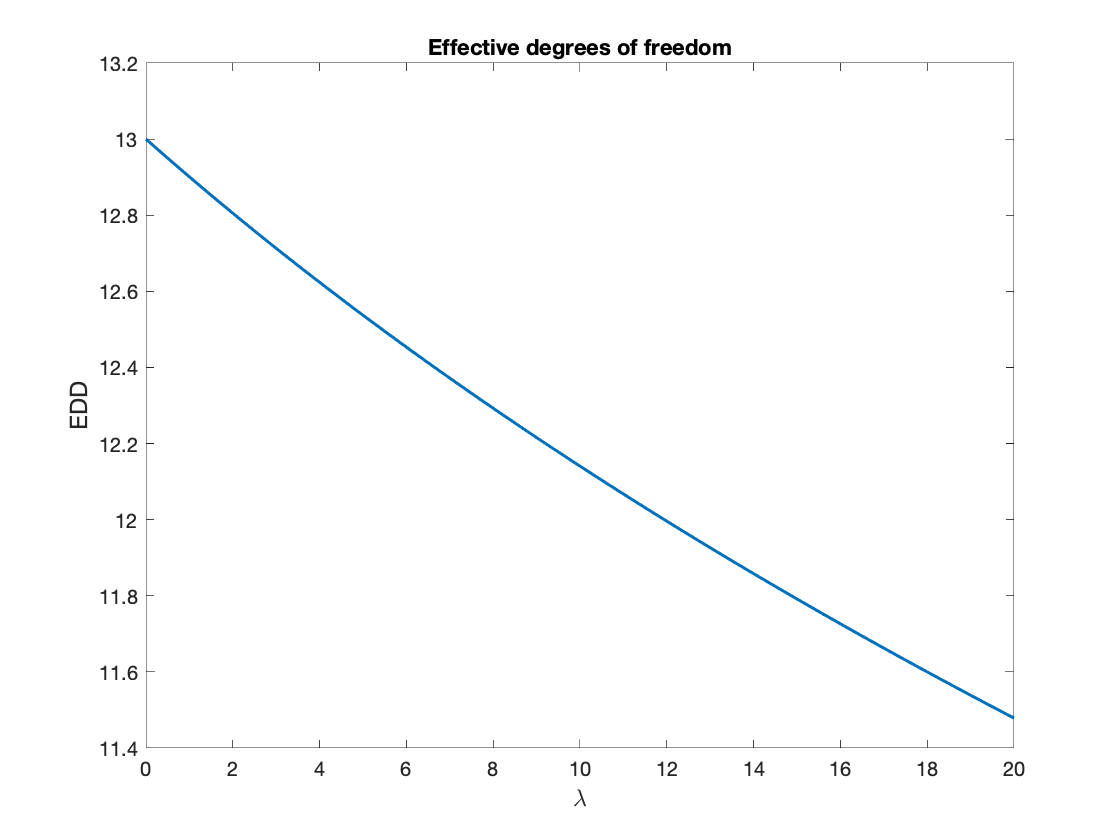Figure 2: Plot Mean-squared prediction error over range.
figure(2)
plot(lambdas, MSE, "Linewidth", 1.5)
title("Mean-squared Prediction Error")
ylabel("MSPE", "FontSize", 12)
xlabel("\lambda", "FontSize", 12)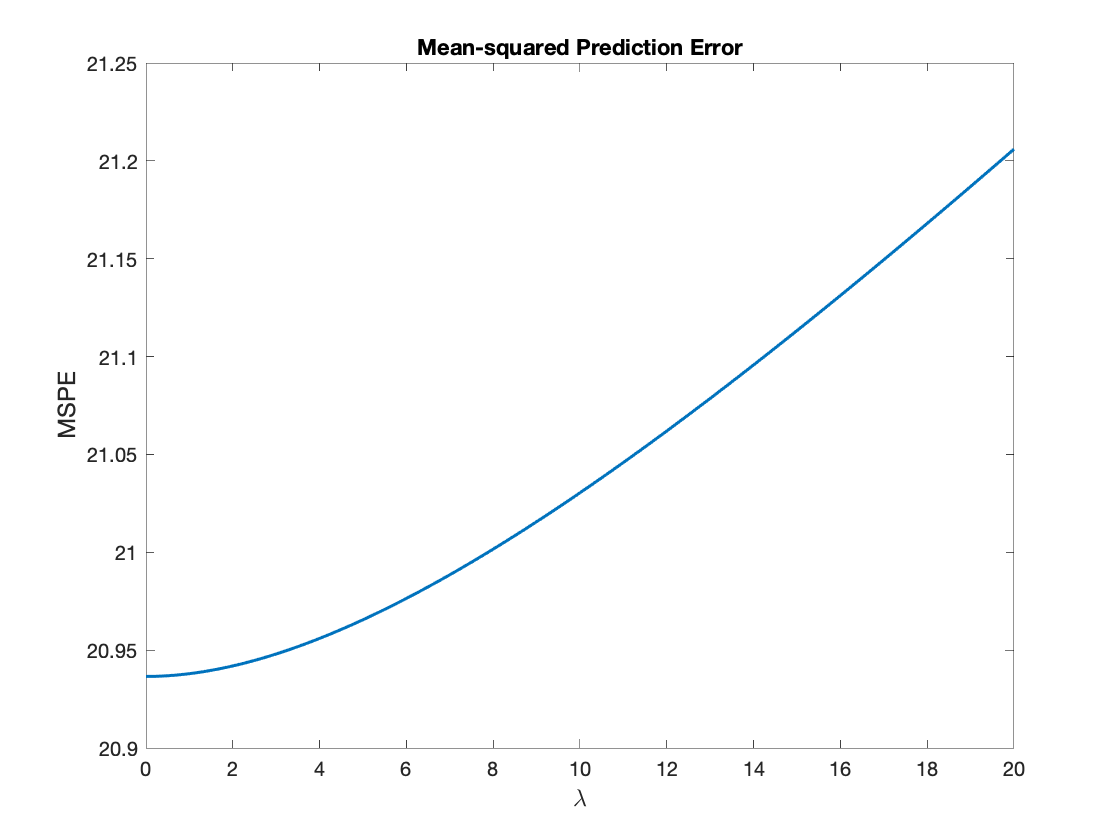% MSPE on hold out set with lambda = 0, i.e. w_train(:, 1)
X_hold = [ones(1, 46); (X(:, subset+1:end) - mu) ./ sigma];
y_hold = y(subset+1:end);
y_hat2 = X_hold' * w_train(:, 1);
MSE_hold_1b = compute_MSE(y_hold, y_hat2);
fprintf(['MSPE on hold-out set with ', num2str(char(955)),' = 0: ',num2str(MSE_hold_1b), '\n'])
MSPE on hold-out set with λ = 0: 27.8722

### c)

(10pts) Now you will use 10 -fold cross-validation on the sphered train-validate samples (see Lec 7 slides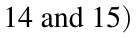to determine the optimal cross-validated value of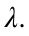Plot the average of the 10 mean kquared prediction errorsthat you found over the cross-validation folds, as a function ofFind the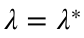that minimizes this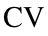error. What is the corresponding effective degrees of freedom associated with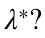Now compute the cross-validated ridge weights wridge that minimizes the PRSS over the entire train+validate set using this value of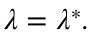Report the average mean squared prediction error of the CV ridge weights when applied to the hold-out set and compare to the error on the hold-out set for the unregularized weightslearned in part b.
MSE_i = zeros(N + 1, 1);
for i = 1:N + 1
% Parameters
lambda = lambdas(i);
MSE_k = zeros(10, 1);
for k = 0:9
X_T = X_sph(:,1:subset);
% Hold out set
X_valid = [ones(1, 46); X_T(:,46*k + 1:46*(k + 1))];
y_valid = y(46*k + 1:46*(k + 1));
% Split set
X_T(:,46*k + 1:46*(k + 1)) = [];
y_T = y(1:subset);
y_T(46*k + 1:46*(k + 1)) = [];
% Compute w_hat, y_hat, MSE
[w_train, ~, ~] = compute_ridge(y_T, X_T, lambda);
% fprintf("The MSE is: " + round(MSE, 3) + "\n")
MSE_k(k+1) = compute_MSE(y_valid, X_valid' * w_train);
end
MSE_i(i) = mean(MSE_k);
end
Figure 3: MSPE 10-fold cross validation
figure(3)
plot(lambdas, MSE_i, "Linewidth", 1.5)
title("MSPE 10-fold cross validation")
ylabel("MSPE", "FontSize", 12)
xlabel("\lambda", "FontSize", 12)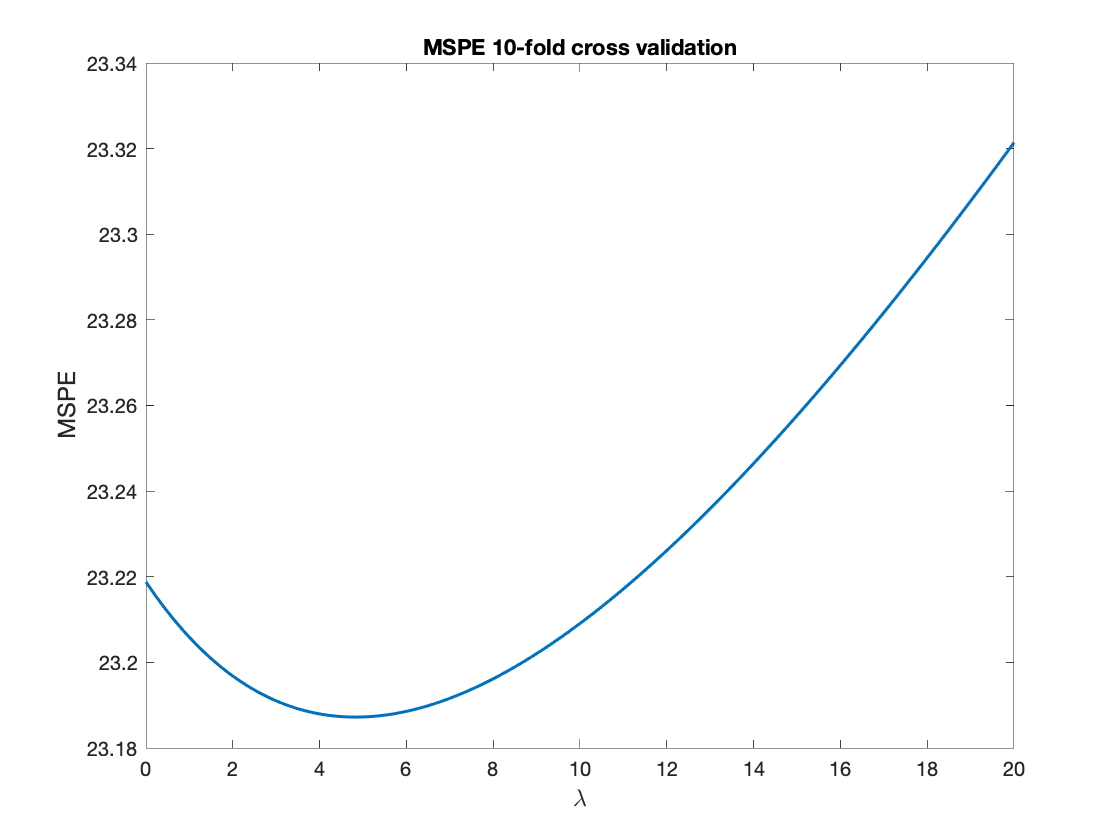Find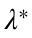and EDD of this:
[~,indx] = min(MSE_i);
lambda_st = (indx - 1) * step;
display(lambda_st)
lambda_st = 4.8000
Display EDD:
EDD_st = compute_EDD(X_sph(:,1:subset), lambda_st);
display(EDD_st)
EDD_st = 12.5544
Compute the cross-validated ridge weights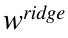that minimizes the PRSS over the entire train+validate set using this value of lambda star
[w_ridge, ~, ~] = compute_ridge(y(1:subset), X_sph(:,1:subset), lambda_st);
disp(w_ridge)
22.5113 -1.0208 1.0195 0.0546 0.5551 -2.0109 2.7969 0.0560 -3.0587 2.3084 -1.8326 -2.0018 0.8795 -3.7053
Compare to hold out set
MSE_hold_1c = compute_MSE(y_hold, X_hold' * w_ridge);
display(MSE_hold_1c)
MSE_hold_1c = 27.7251
fprintf(['The MSPE on hold-out set with ', num2str(char(955)),' = ',num2str(lambda_st), ...
' and CV ridge weights is ', num2str(MSE_hold_1b - MSE_hold_1c), ' smaller than ', ...
'MSPE without regularization.'])
The MSPE on hold-out set with λ = 4.8 and CV ridge weights is 0.14703 smaller than MSPE without regularization.

## 2) Descent methods for regression (15pts)

### a)

(7 pts) Implement the (batch) gradient descent (GD) update rule to train your regression model on the training data. Set the initial weight to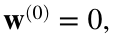the step size parameter to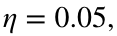and the number of GD iterations to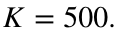Plot the training error as a function of iteration and report the learned weight vector obtained after K iterations. Evaluate the mean squared prediction error of the learned weight vector when reapplied to the entire training sample and when applied to the test sample. Compare the learned GD weights to the directly computed weightslearned from the training data.
K = 500;
eta = 0.05;
w_k = zeros(d + 1, 1);
train_error = zeros(K, 1);
% Perform Batch GD
for j = 1:K
k = mod((j - 1), 10);
% Get batch of X_j and y_j
X_j = [ones(1, 46); X_sph(:,46*k + 1:46*(k + 1))];
y_j = y(46*k + 1:46*(k + 1));
g = (2 / n) * X_j * (X_j' * w_k - y_j);
w_k = w_k - eta * g;
y_hat = X_j' * w_k;
train_error(j) = compute_MSE(y_j, y_hat);
end
Figure 4: Plot the train error as a function of iteration
figure(4)
plot(train_error, "Linewidth", 1.5)
title("Train error as function of iteration, Batch GD")
ylabel("MSPE", "FontSize", 12)
xlabel("kth iteration", "FontSize", 12)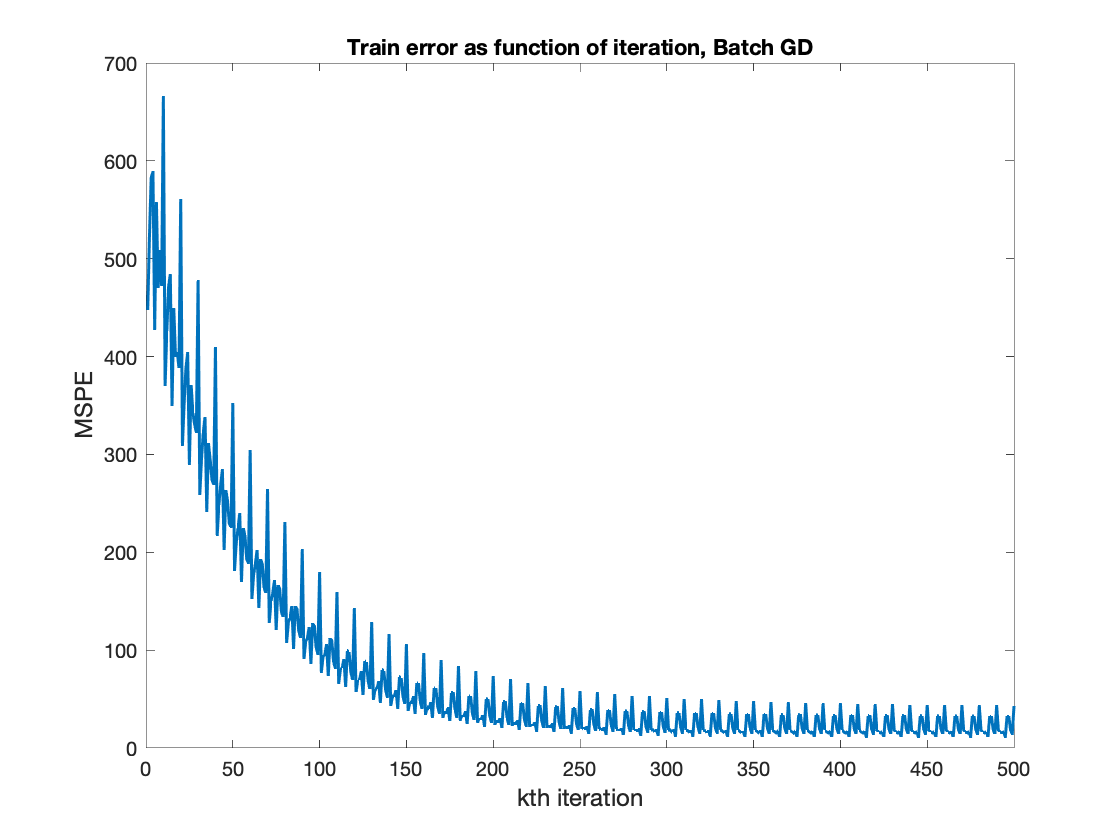Report final learned weight vector
w_GD = w_k;
disp(w_GD)
22.2902 -0.7859 0.5658 -0.3961 0.6295 -1.0096 3.2500 -0.0843 -2.1139 0.7817 -0.4815 -1.8390 0.9278 -3.4638
Evaluate the mean squared prediction error of the learned weight vector when reapplied to the entire training sample and when applied to the test sample.
y_hat = [ones(1, subset); X_sph(:, 1:subset)]' * w_GD;
MSE_train_GD = compute_MSE(y(1:subset), y_hat);
display(MSE_train_GD)
MSE_train_GD = 21.9025
MSE_test_GD = compute_MSE(y_hold, X_hold' * w_GD);
display(MSE_test_GD)
MSE_test_GD = 28.3228
Compare the learned GD weights to the directly computed weights learned from the training data. We show the difference between estimates of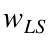and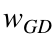:
[w_LS, ~, ~] = compute_ridge(y(1:subset), X_sph(:, 1:subset), 0);
compare_w = w_LS - w_GD;
disp(compare_w)
0.2211 -0.2787 0.5350 0.5775 -0.0902 -1.1463 -0.4896 0.1689 -1.0938 1.8941 -1.7053 -0.1985 -0.0466 -0.3065

### b)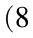pts) Implement stochastic gradient descent (SGD) to train your regression model. Set the initial weight to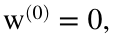the step size parameter to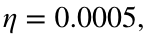and the number of SGD epochs to ortorAs in a, plot the training error as a function of epoch and report the learned weight vector obtained after K epochs. Evaluate the mean squared prediction error of the learned weight vector when reapplied to the entire training sample and when applied to the test sample. Compare the learned SGD weights to the GD weights, and to the directly computed weights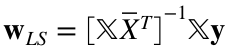learned from the training data.
eta = 0.005;
w_k = zeros(d + 1, 1);
train_error = zeros(K, 1);
% Perform SGD by epochs
for epoch = 1:K
rand_j = randperm(10) - 1;
for i = 1:10
k = rand_j(i);
% Get batch of X_j and y_j
X_j = [ones(1, 46); X_sph(:,46*k + 1:46*(k + 1))];
y_j = y(46*k + 1:46*(k + 1));
g = (2 / n) * X_j * (X_j' * w_k - y_j);
w_k = w_k - eta * g;
y_hat = X_j' * w_k;
end
train_error(epoch) = compute_MSE(y_j, y_hat);
end
Figure 5: Plot the train error as a function of K epochs
% Plot the training error as a function of epoch
figure(5)
plot(train_error, "Linewidth", 1.5)
title('Train error as function of K epochs')
ylabel("MSPE", "FontSize", 12)
xlabel("kth epoch", "FontSize", 12)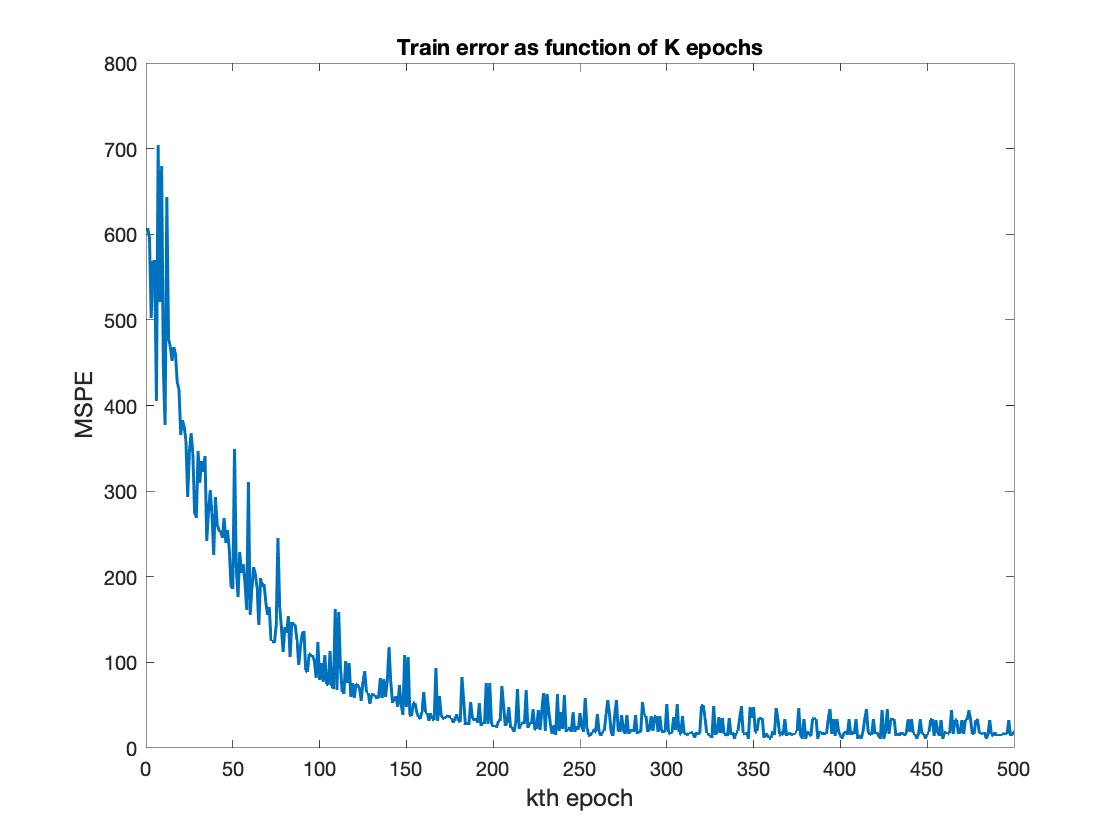Report the learned weight vector obtained after K epochs.
w_SGD = w_k;
disp(w_SGD)
22.2731 -0.7861 0.5606 -0.4056 0.6228 -1.0098 3.2291 -0.0858 -2.1063 0.7797 -0.4893 -1.8375 0.9284 -3.4522
Evaluate the mean squared prediction error of the learned weight vector when reapplied to the entire training sample and when applied to the test sample.
y_hat = [ones(1, subset); X_sph(:, 1:subset)]' * w_SGD;
MSE_train_SGD = compute_MSE(y(1:subset), y_hat);
display(MSE_train_SGD)
MSE_train_SGD = 21.9126
MSE_test_SGD = compute_MSE(y_hold, X_hold' * w_SGD);
display(MSE_test_SGD)
MSE_test_SGD = 28.3397
Compare the learned SGD weights to the GD weights by taking differences of the estimates.
compare_w_SGD_GD = w_SGD - w_GD;
disp(compare_w_SGD_GD)
-0.0171 -0.0003 -0.0052 -0.0094 -0.0067 -0.0002 -0.0209 -0.0015 0.0075 -0.0019 -0.0078 0.0015 0.0006 0.0117
Compare SGD weights to the directly computed weights learned from the training data by taking differences of the estimates.
compare_w_SGD = w_LS - w_SGD;
compare_w_SGD_GD = w_SGD - w_GD;
disp(compare_w_SGD)
0.2382 -0.2784 0.5402 0.5869 -0.0836 -1.1460 -0.4687 0.1704 -1.1013 1.8961 -1.6975 -0.2000 -0.0472 -0.3182

## 3) Subgradient methods for the optimal soft margin hyperplane (25 pts)

### b)

Implement the subgradient method for minimizing J and apply it to the nuclear data. Submit two figures: One showing the data and the learned line, the other showing J as a function of iteration number. Also report the estimated hyperplane parameters and the minimum achieved value of the objective function.
clear
clc
% Implement the subgradient method for minimizing J and apply it to the nuclear data.
% Submit two figures.
rng(0);
% Initialize parameters
K = 100;
lambda = 0.001;
[d, n] = size(x);
x = [ones(1, n); x];
theta_k = ones(d + 1, 1);
D = diag([0; ones(d, 1)]);
J = zeros(K, 1);
% f = waitbar(0, 'Please wait...');
% Iterate K times to update theta_k
tic;
for j = 1:K
indic = theta_k' * x * diag(y);
g = -(1/n) * x * (y' .* (indic < 1)') + lambda * D * theta_k;
alpha_j = K / j;
theta_k = theta_k - alpha_j * g;
J(j) = compute_J(y, x, theta_k, lambda);
% waitbar(j / K, f, sprintf('Trial %d of %d', j, K));
end
time_GD = toc;
We obtain the learned line from: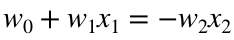.
data = [ones(n, 1), linspace(0, 7, n)'];
pred_line = -(1/theta_k(3)) * (data * theta_k(1:2));
Figure 6: Showing the data and the learned line
figure(6)
scatter(x(2, y == 1), x(3, y == 1))
hold on;
scatter(x(2, y == -1), x(3, y == -1))
plot(linspace(0, 7, n)', pred_line, 'LineWidth', 2)
title('Data and Learned line')
xlabel('Total energy', "FontSize",12)
ylabel('Tail energy',"FontSize",12)
hold off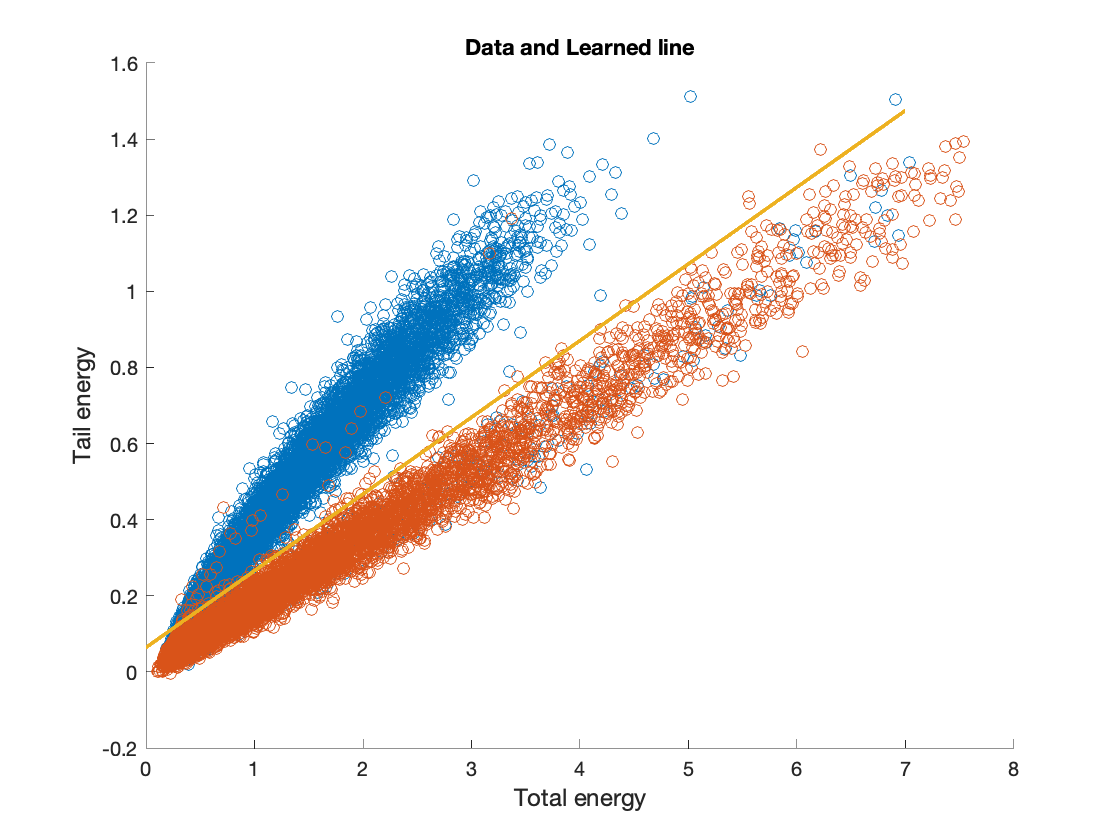Figure 7: Showing J as a function of iteration number.
figure(7)
plot(J, 'Linewidth', 1.5)
xlabel("kth iteration", "FontSize",12)
ylabel("$J(w,w_0)$", "FontSize", 12, "Interpreter","latex")
title('J as function of iteration with subgradient method')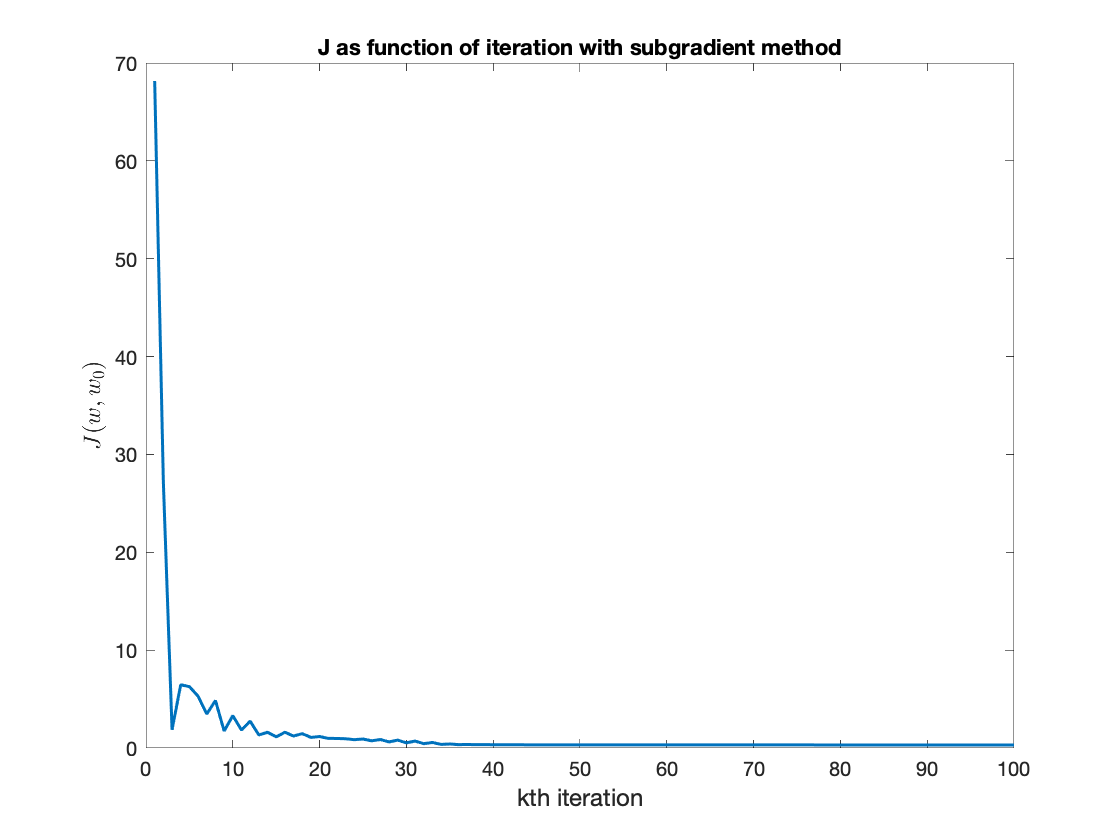Report the estimated hyperplane parameters and the minimum achieved value of the objective function.
hyper_p = theta_k;
display(hyper_p)
hyper_p = 3×1
-0.9927 -3.1202 15.4776
min_obj = min(J);
display(min_obj)
min_obj = 0.3105

### c)

(6 pts) Now implement the stochastic subgradient method, which is like the subgradient method, except that your step direction is a subgradient of a random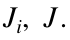Be sure to cycle through all data points before starting a new loop through the data. Report/hand in the same items as for part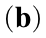.
rng(0);
% cycle K times through theta_k
% f = waitbar(0, 'Please wait...');
cycle_size = 10;
J = zeros(cycle_size * K, 1);
tic;
for j = 1:K
% Set up permutations
rand_j = randperm(cycle_size) - 1;
% Step-size
alpha_j = K / j;
% Begin Cycling through data
for i = 1:cycle_size
k = rand_j(i);
subset = n / cycle_size;
x_j = x(:,subset*k + 1:subset*(k + 1));
y_j = y(subset*k + 1:subset*(k + 1));
indic = theta_k' * x_j * diag(y_j);
g = -(1/n) * x_j * (y_j' .* (indic < 1)') + lambda * D * theta_k;
theta_k = theta_k - alpha_j * g;
J(cycle_size * (j - 1) + i) = compute_J(y_j, x_j, theta_k, lambda);
end
% waitbar(j / K, f, sprintf('Trial %d of %d', j, K));
end
time_SGD = toc;
We obtain the learned line from:.
data = [ones(n, 1), linspace(0, 7, n)'];
pred_line = -(1/theta_k(3)) * (data * theta_k(1:2));
Figure 8: Showing the data and the learned line
figure(8)
scatter(x(2, y == 1), x(3, y == 1))
hold on;
scatter(x(2, y == -1), x(3, y == -1))
plot(linspace(0, 7, n)', pred_line, 'LineWidth', 2)
title('Data and Learned line')
xlabel('Total energy', "FontSize",12)
hold off
ylabel('Tail energy',"FontSize",12)